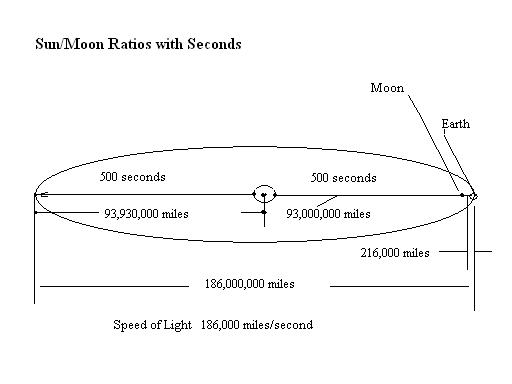David Jay Jordan's                                                                                                                  Home -  Math

Sun Moon Distance Ratios with Seconds

The Sun is the center of the Solar System which is the center of the Universe. And the Earth is where the Lord choose to reveal
Himself for All His Creation. So lets look and see if there is a correspondence of distance with time in His Harmonic Design of His
Tabernacle of the Sun/Son.

First of all we now know, are 93,000,000 miles away from the Sun, although this varies slightly because our orbit is slightly
elliptical. And as we found out our orbit means that His light to us, takes 500 seconds to reach us, or takes 1000 seconds to go
across our orbital plane from one side to the other. Why because the Lord made light speed to match this distance to us, and hence
light speed was set by Him at 186,000 miles per second. 186,000,000 miles being the diameter of the Suns Tabernacle orbital circle.
And 93 being the radius number and  of 186.

So similarly in feet, light speed is 1,000,000,000 feet per second, or 1 foot in a nanosecond. This being important because his
creative light speed that was first set, had to be slowed down in correspondence to the speed of His Voice here on Earth, as Hi
words created all life. The speed of sound being at approximately 1,000 /per second rather than light speed of 1,000,000,000/per
second a step down factor of 10 to the sixth, or  light travels 1,000,000 times as fast as sound. (SEE also Creation of the Elements)

But let us see if our daily revolution speed corresponds in anyway to this distance from the Sun. Our revolution of the Earth being
once every 24 hours. But in seconds this is 24 x 60 x 60 = 86,400 seconds. The number of seconds in an equinox day being half of
this or 43,200.

Hmmmm¦ but there is a factor number in the range of 432. There is of course, the human voice tuning frequency of A at 432 hertz.
And 432 is the ratio distance between the Moon and the distance of the Sun from us. Why is the Moon important, well it is called
the Bride, the feminine that will be united with the Bridegroom the Son or Sun. And as Enoch stated they are equal, just as in the
piezoelectricity of the hexagonal triangles of male and female. (SEE
Sexy Star of David and Geometry of Crystals).

But the problem is the Moon is 216,000 miles from us and 2160 miles in diameter, whereas the Sun is 93,000,000 miles from us and
has a diameter of 930,000. SEE Graphics â€¦. And hence there ratio of difference is 93,000,000/216,000 = 430.555¦

But then again we are going from the surface of the sun, as the distance to us, when in fact, its light come from its center, so lets add
the radius of the sun to its distance from us. Therefore 930,000/2 equals 465,000. Adding this to 93 million miles gives us ¦
93,465,000 miles¦ and now lets do that comparison of ratios (knowing the Moons light to us comes from its surface rather than
from its core. â€¦as we, the bride merely reflect the Bridegrooms light)

93,465,000/216,000 =  432.734¦. And we are close enough to exactly 432 as the numbers surely are in exact correspondence the
more we find out the true exact ratio measurements¦..

So in my opinion mathematically, just as the Moons diameter was set in proportion to the Earths diameter to make a perfect PHI
Pyramid when the MOON Comes down to the Earth. (SEE Magic ratios), so also the distance of the Moon and Sun from us,
determined the Lords Timing for us. He created the boundary and speed of Light, and then set us the appropriate distances away so
that his Light would reach us in 500 seconds, just as 500 feet is the height of His Great Pyramid in Giza, the Cydonia mound,
Glastonbury Tor, and a multiple of His Great Eternal City, New Jerusalem, which is 10,000 times the size of the Great Pyramid.

And then the ratio of the distances of  the Moons distances from us over the distance to the Sun gives us the 432 factor which times
by 100 gives us the number of seconds we are allowed in a day. Coincidence, I think not, as the Lords ratios, distances, speeds, and
times are all too exact and all are harmonic together !!

Dont you agree !!

David